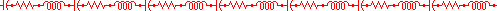# V73NS## Calculations to Design Your Own Antennas

Ground Planes

5/8th Wave Vertical

Dipoles and Inverted Vees

### Ground Planes

The standard ground plane antenna consists of a vertical radiator which is insulated and centered between 4 horizontal surrounding radials which extend out from the base of the antenna. Each radial is evenly spaced 90 degrees or so from each other around the vertical element.
Gain is -0.29 dB less than dipole. Angle of radiation is 27 degrees.

Enter the formula for the antenna calculation (normally 234/f):

divided by Freq. MHZ

The vertical length is feet.

The 4 radials are each feet.

(This is same formula for any full wave loop, such as a Delta too)
The cubical quad antenna is a four sided antenna, with each side a quarter wave length long.  Several factors influence the performance of the antenna, such as :
• The antenna height above the ground
• The diameter of the wire used
• Nearby structures to where the antenna is mounted
• Effects of other antennas or metal objects in the area
• The conductivity of the soil
Enter the formula for the antenna (normally 1005/f):
divided by Freq. MHZ

Each of the 4 sides are feet.

### 5/8th Wave Vertical Antenna

The 5/8 wave antenna consists of a vertical radiator  fed at the bottom of the antenna. A matching device should be used if you feed it with coax.
Gain is 1.87 dB over a dipole. Angle of Radiation is 16 degrees.

Enter the formula for the antenna (normally 585/f or 178.308/f for metric):
Switch to:

divided by Freq. MHZ
Your 5/8 wave antenna's Vertical element is

### Dipole and Inverted Vee Antennas

Most familiar are the centerfed, halfwave dipoles and inverted vees that are so popular.
A basic formula for these types of antennas is below.  For center fed, wire dipole antennas, the formula is 468/freq(mhz).
Enter the formula for the antenna:

divided by Freq. MHZ

Percent smaller for an Inverted Vee.
Your dipole's total length is feet. Each leg of the dipole is feet.
Your Inverted Vee's total length is feet. Each leg of the Inverted Vee is feet.

last updated on 27-Sep-2015